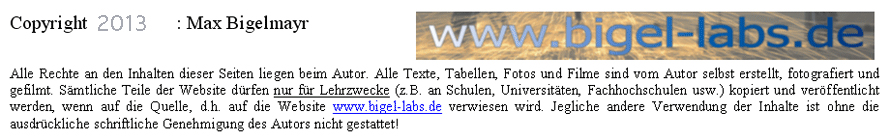Physics

Theoretically Applied Electrodynamics

Some thought's on the "Russian Capacitors"
...with regard to the newly planned capacitor bank...

 1. Analysis of the pulse capacitors 2. Discharge of the entire capacitor bank 3. Is it possible to use the capacitor at I(max(>3kA with a lower pulse rate than R=12/min? 4. Discharge of the entire capacitor bank- pulse magnetic forming/welding 5. Discharge of the entire capacitor bank- Exploding wires .. Abstract: On this particular page I want to discuss the discharge behaviour of the Russian Capacitors, which we are planning to use in a large capacitor bank.This capacitor bank will be able to store around 40.000 Joules of energy at 12.000 volts. Also watch Stefans page: http://www.teslathon.de/stefan/tc/joule_MP.htm Max Bigelmayr, October 2011, April 2013

#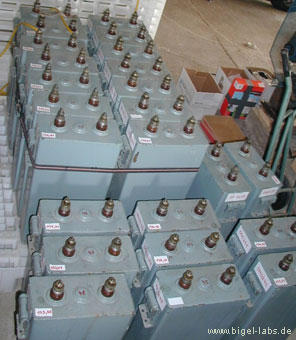1. Analysis of the pulse capcitors

´We have 36 pulse capacitors to our disposal. After translating the original data sheets from russian into english, the following information was given:

 nominal Voltage: 5kV Capacitance: 140uF ESL: <600nH Discharge pulse rate: 10-12/min max. Current: 2,5-3kA log. Dekrement: 2-2.5

Datasheet of the capacitors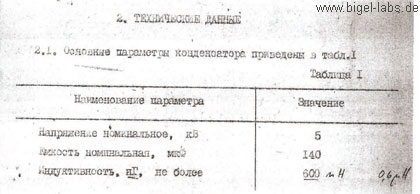Within the capacitor bank, individual electrical condensers will be connected to one another.
To analyse the discharge behaviour, I programmed a special "Capacitor-Analysing-File".
With the help of this program, I was able to solve the differential-equation-system by using Runge Cutta Algorithms, which provided me with the numerical discharge curves, concerning U(t), I(t), A(t).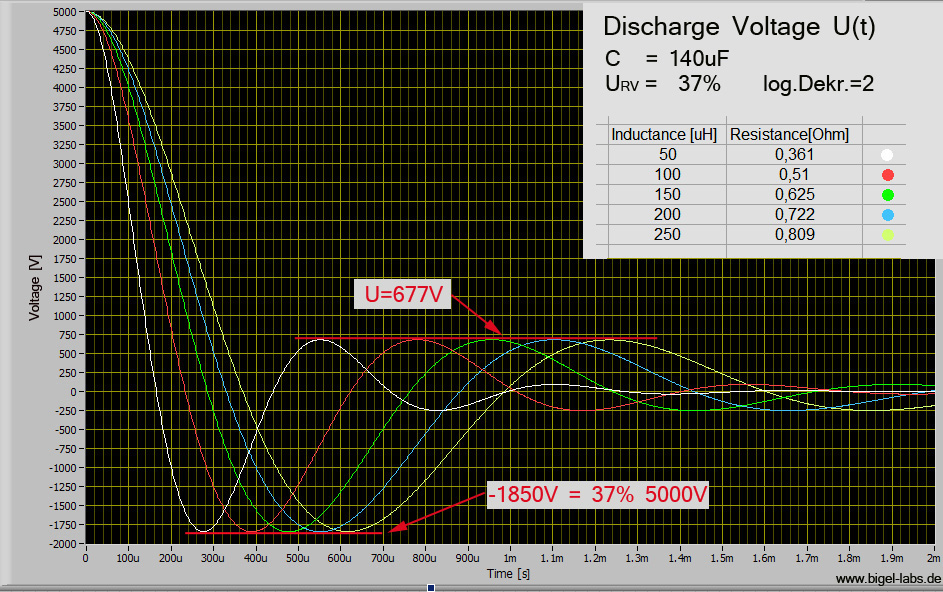Graph 1:
The parameters L and R have been specifically chosen, so that all curves have a reversal voltage of -1850V, which is equal to a log. Dekr.=2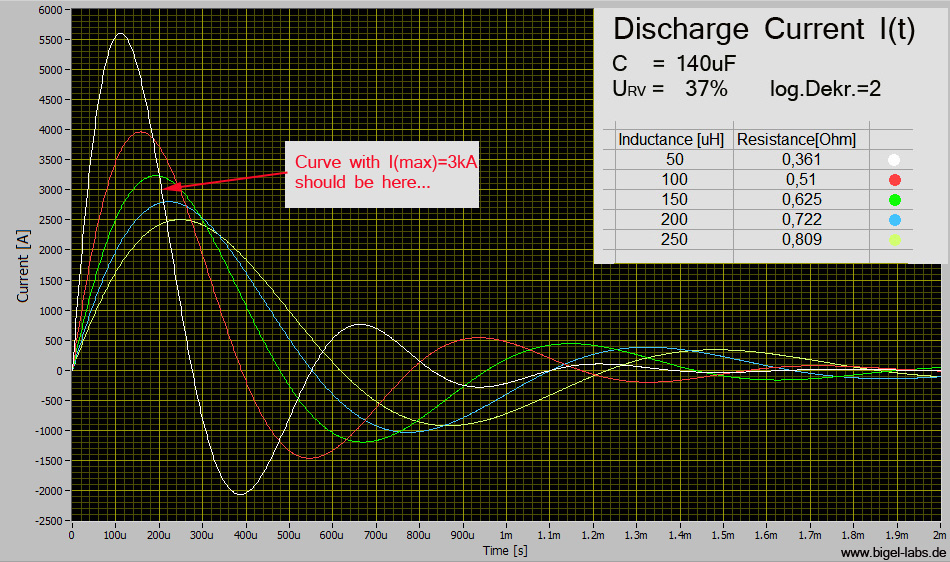Graph 2:
Currents I(t) with the same parameters as before

I tried to find the curve with Imax= 3kA and a reversal voltage of 37%. It´s a CLR circle with C=140uF, L=175uH and R=0.65Ohm.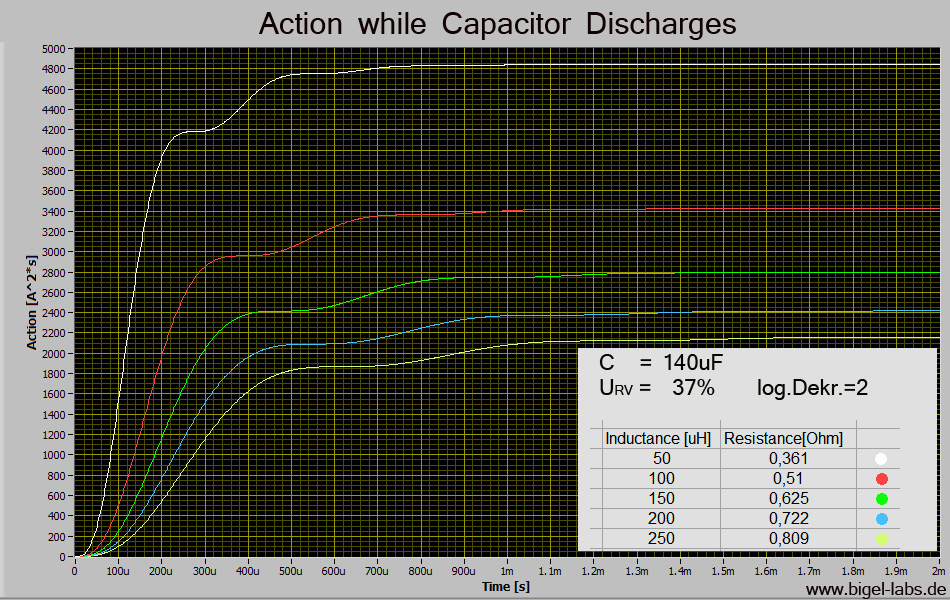Graph 3:
Action curves A(t) with the same parameters as before

2. Discharge of the entire capacitor bank

The russian capacitors ought to be used within a new capacitor bank: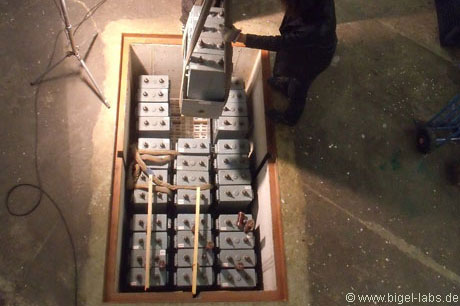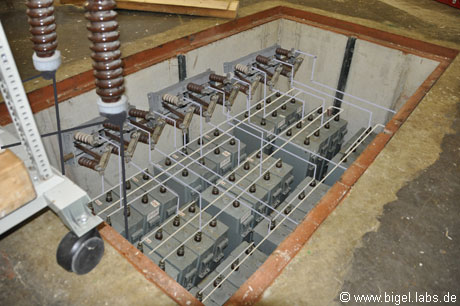Installation of the Capacitor bank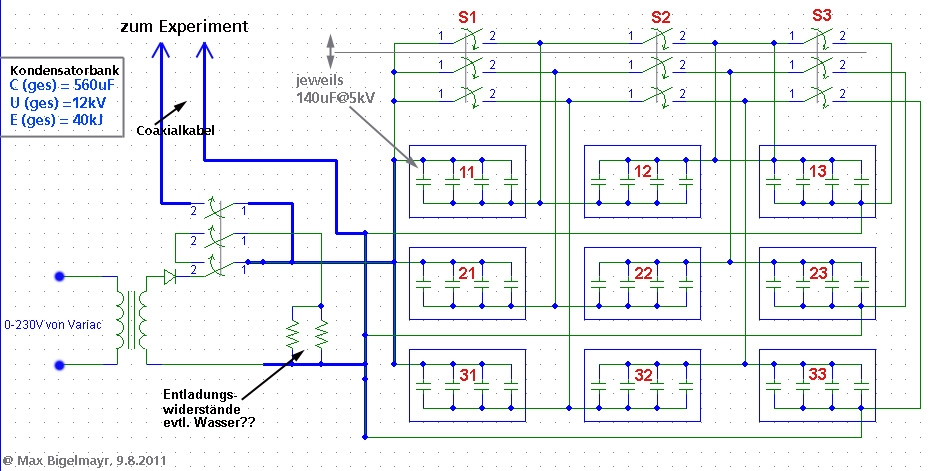Let us assume that a pulse capacitor bank network, with n identical capacitors which are joined together in parallel is assembled.
If we neglect the inductance between the capacitors, we can assume that I1=I2=I3....In.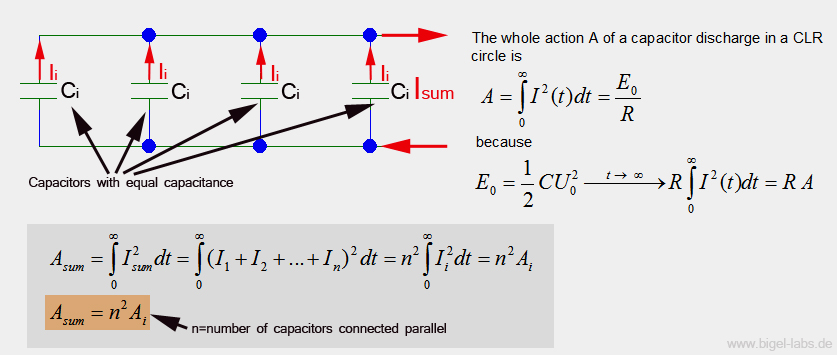In the planned capacitor bank, we will connect 12 capacitors in parallel (view schematic diagram above). Prior to this we found out that the maximum Action A for one capacitor is 2600A^2s. Now it is very easy to calculate Asum: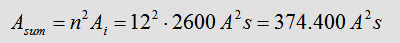The total capacitance of the capacitor bank will be situated at 560uF. The stored energy of 40kJ at 12kV, allows for the calculation of the minimal load resistance permitted during pulse-experiments. In general we can say: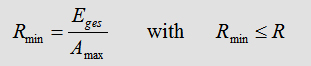For the used capacitor bank Rmin is: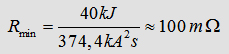In the following step it is possible to simulate different discharges of the capacitor bank with a defined R=100mOhm: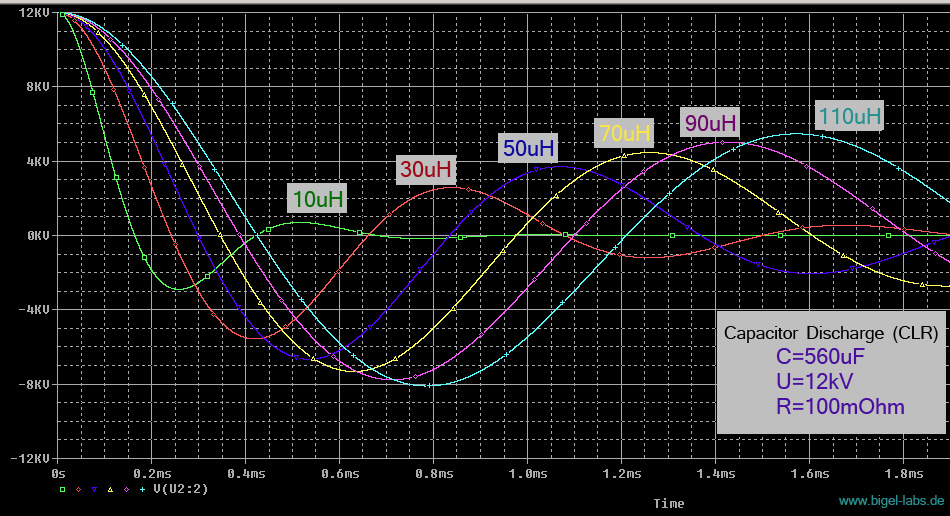Graph 4: Discharge Voltage Uc(t) of the entire capacitor bank with a different line inductance and a load resistance of 100mOhm.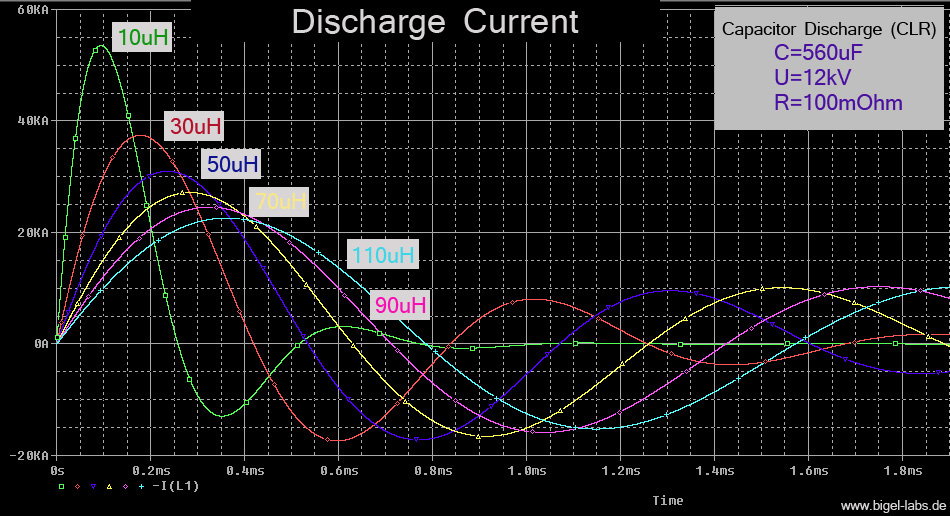Graph 5: Discharge current Isum(t) of the entire capacitor bank with a different line inductance and a load resistance of 100mOhm.

3. Is it possible to use the capacitor at I(max(>3kA with a lower pulse rate than R=12/min?Earlier we calculated the maximum Action Integral of one of the
russian capacitors: A=2600A^2s. With this value in mind we are able to reflect on the internal fuse within the capacitors. Let us assume that it is a specific type of iron wire. The wire should be the critical part, which warms up during discharge. Every discharge-pulse will cause for the wire to heat up. If the capacitor is used every 5 seconds, in a pulse-performance mode (pulse rate of 12pulses/min), the capacitor is charged and discharged in sudden succession. The action integral A(charge) regarding the charge, is precisely equal to the action integral A(discharge) concerning discharge.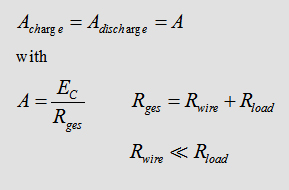First of all the capacitor is charged up within 5s. We could use a source of voltage with a current of U0=5kV. The voltage and current are described by the following: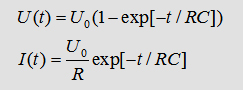It is fairly straight forward to calculate the Action-integral and the limes for time-> infinity:However we haven't got an endless amount of time to disposal. Therefore a typical load circle/cycle?? could be a voltage source with a current of U=6kV, followed by an Ohm-resistor. Thus the voltage will reach precisely 5kV after 5s.4. Discharge of the whole capacitor bank- pulse magnetic forming/welding
[ supplement April 2013]

ome pulsed power experiments with spiral coils. With the capacitor bank KB1-KB2 I achieved discharge pulses similar to the following: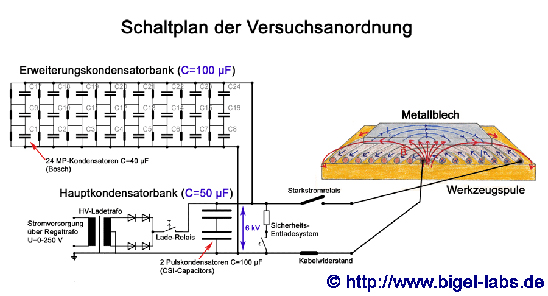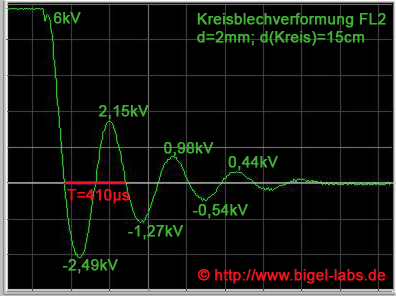Schematics of the capacitor bank KB1-KB2 Capacitor voltage U(t) while a discharge with Spiral coil FL2

One may try to simulate the discharge behavior with PSpice to get a similar voltage curve U(t). I tried many different Schmematics and values to describe be measured curve U(t). One possible circuit is this: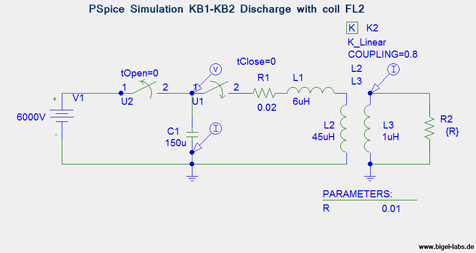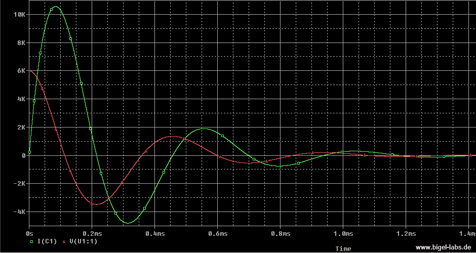PSpice Simulation of the capacitor bank KB1-KB2 Simulation of Capacitor voltage U(t) and Discharge Current I(t) with Spiral coil FL2

In this simulation the current grows up to around 10,5kA peak.
If we use the large 40kJ capcitor bank instead of the "small KB1-KB2" one may assume a line inductance of around 20uH. Let´s use the coil FL2 and subsitute the capacitance with 560uF and the voltage source with 12.000V. In this case the circuit becomes: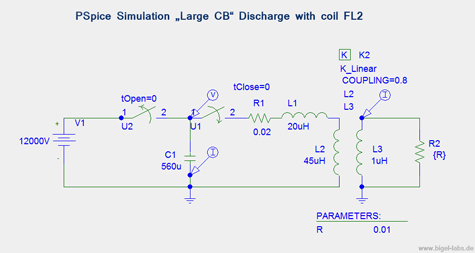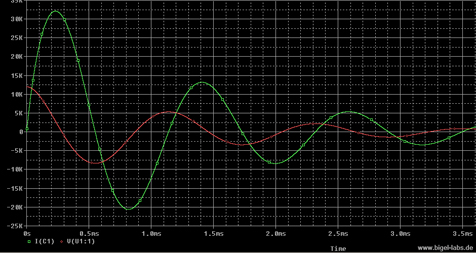PSpice Simulation of the Large Capacitor Bank (LCB) Simulation of Capacitor voltage U(t) and Discharge Current I(t) with Spiral coil FL2

You may realize the bad damping while the discharge. The reversal voltage is around 8kV, which is equal to 8kV/12kV=2/3 ~> 66% One may also calculate the quality factor Q with the voltage reversal %RV: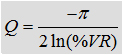With RV=66% the quality factor becomes -0.37. The stresses on capacitors caused by reversal include increased electric fields in the dielectric, increased electrical losses, heating in the dielectric and heating in the conductors. In many studies GENERAL ATOMICS ENERGY PRODUCTS found out that the life time of capacitors may be pushed by derating the voltage reversal. As we calculated in the beginning the nominal voltage reversal of the russian capacitors is around 37%. For lifetime reasons the capacitor bank will be only discharged at a voltage level of 80%Umax(nom). So each individual capacitor will be charged up to only 4kV instead of 5kV. Let´s discuss how this technic does influence the lifetime coefiicient.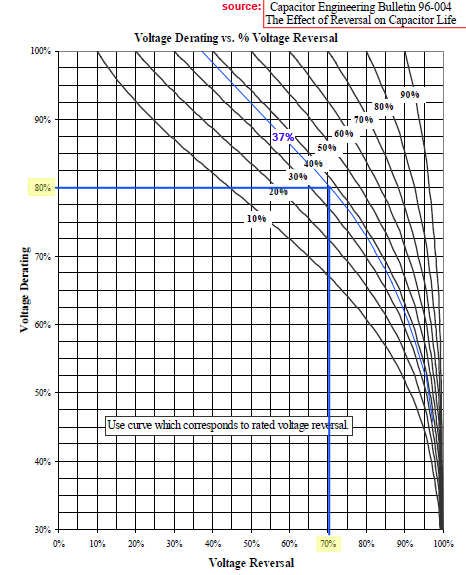With the graph on the left sight it´s possible to find a new reversal voltage for a specific capacitor if you know the reveral voltgage by nominal voltage use. In our case we have got a nominal Voltage Reversal of 37% at 5kV. So we will get a "new reversal voltage" if we only charge up to 4kV, which is a Voltage Derating of 80%. In this case we get a new Voltage Reversal of around RV=70%. That´s really a nice value, isn´t it?? ;-)
With the graph on the right we may existimate the Life Expectancy Multiplier (LEM). With the coil FL2 (RV=66%) the LEM is around 1.2-1.3, so that we would get an "pushing life factor".
The coil inductance (L=45uH) is just around two times the line inductance (L=20uH). While the discharge the energy may only/manly be absorbed in the coil. While the maximum current of I=32kA we have got a capacitor voltage of around 3.75kV. At this time the energy distribution is: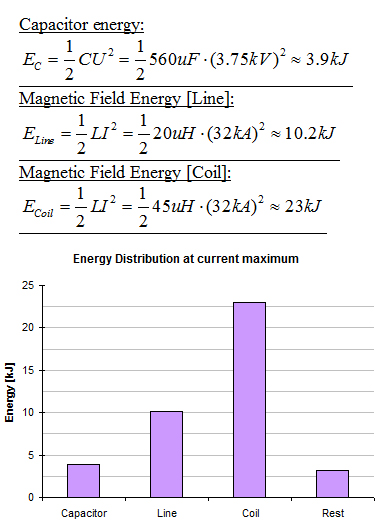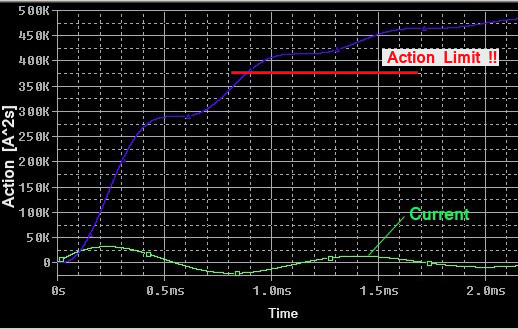Simulation of the Action Integral while the discharge As we saw before the voltage reversal of around 66% is not really hard to the capacitors. But what about the Action integral? It´s growing up to high!!   So, what´s to do??

There are three possible solutions:
1.) Using of "high inductance coils"
We could use high inductance coils with L >100uH to maximize the quotient L(coil)/L(ges). This will give a much better damping and low voltage reversal. The disadvantage is a low currentraise dI/dt. The metals we want to form won´t be accelareted that fast with "high inductance coils".
2.) Using of parallel diodes
We could use high voltage high current diodes.
We haven´t got such diodes. Maybe it is possible to use cheap high voltage diodes (with low current rates) and vaporize them while the discharges.
3.) Using of vaporization coils
By using coils with a low wire diameter ist´s possible to find a perfect wire diameter, so that the coil will vaporize just after the first half wave. In this case the capacitors don´t have to absorb all the low damping oszillation energy.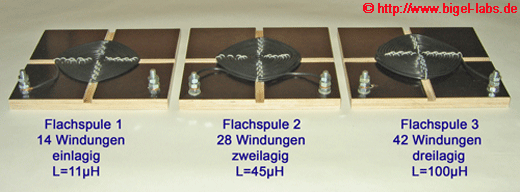Simulation with "high inductance coils"
In 2006 I also used a three layer spiral coil with 42 roundings and a inductance of around 100uH (picture on the right).

A simulation with this inductance gives us the followinhg discharge characteristic...5. Discharge of the entire capacitor bank- Exploding wires

The discharge behaviour of capacitor banks, through a variable resistor R(t) is a fairly complicated topic. To understand this phenomena, I programmed a tool with which I am able to input and analyze all the required data (capacitance, voltage, load inductance, wire material, diameter, length...)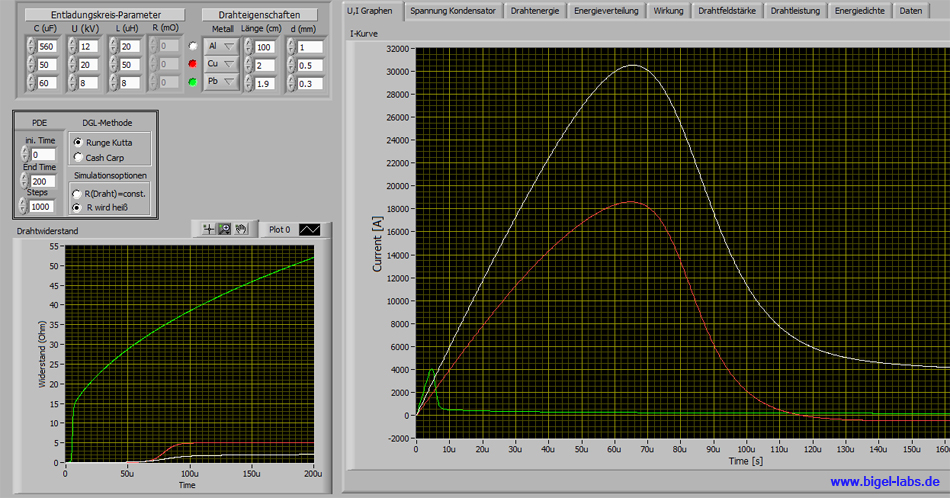With the program I can simulate: - discharge currents - discharge voltages - field strenght inside the wire - energy density in the wire - absorbed energy in the wire - power curves - periodic times - Action integral .....................................and a lot moore.... The coupled differential equation systems are solved by using Runge Kutta or Cash Carp algorithms. It is possible to compare different wires in one graph. The new capacitor bank should be used with an Action-integral of less than 375.000A^2s. With the give simulations, one is able to discover the optimum parameters concerning such a pulse discharge. First theory, after that experiments.......

Simulation results for these Parameters: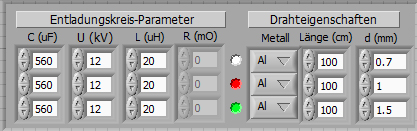.....Selecet the graphs you want to watch with the mouse.....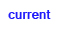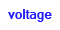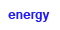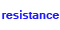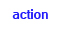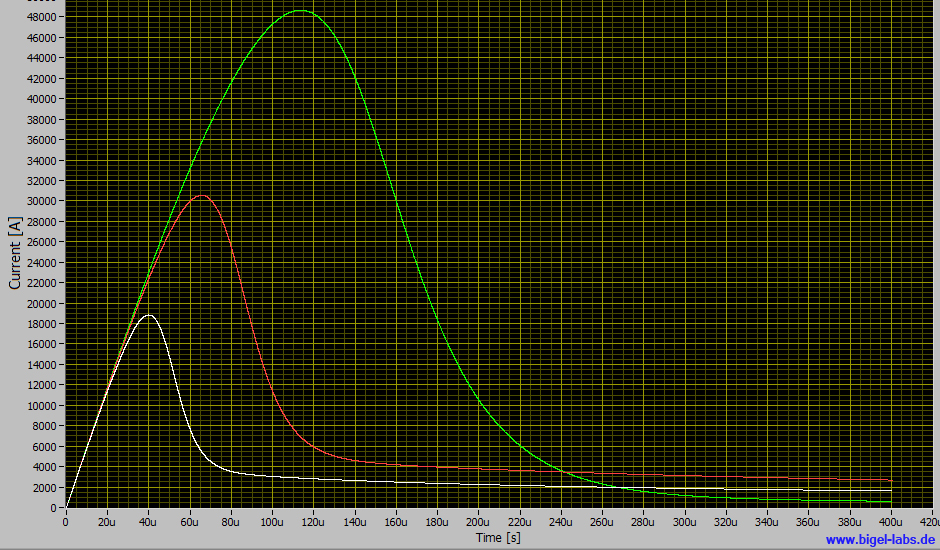In all three cases the action integral is lower than 375kA^2s. With a diameter of 1.5mm, the action integral would achieve 225kA^2s.
In real experiments the wire vaporizes, resulting in a very rapid breakdown of the current. Most experiment's will occur as puls-discharges. During the first pulse the wire is heated up. The resistance of the wire suddenly prevents a discharge. This occurrence is known as a darkness brake. Following this phenomena, the metal boils and "metal gases" leave the wire in a radial manner. This resulting plasma surrounding the wire, will initiate a second discharge pulse. In general one can assume that all these effects will not create a larger action integral than calculated in the simulation above. Therefore such experiments are possible without the fear of killing the capacitors through such discharges.